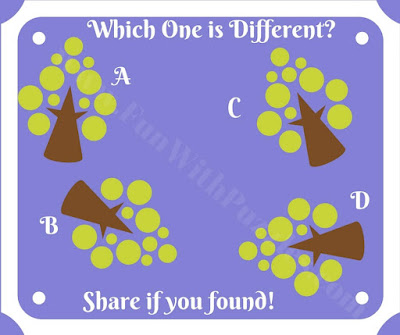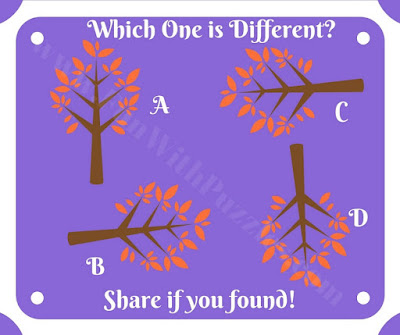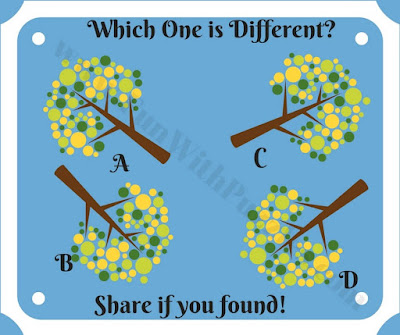## Tuesday, March 17, 2020

There are many different picture puzzles on this website. There are picture puzzles in which one has to find a mistake in given pictures. There are puzzles in which one has to find which number is different from other given numbers. There are puzzles in which one has to tell which alphabet is different from other given alphabets. There are picture puzzles to solve parking slots in which one has to tell where the particular vehicle has been parked which can be deducted from looking at the sequence of parking numbers which are still not parked.  Also, there are picture puzzles with hidden elements such as hidden animals picture puzzles in which one has to find the hidden animals in the given pictures. Also, there are puzzles in which one has to find out a hidden letter in the groups of given letters. Today's picture puzzles are based upon Odd One Out genre.
In these puzzles, four pictures are given which looks exactly alike. However, there is one minuscule but significant difference in one of the pictures which makes that picture different from the other three pictures. Your challenge is to find this difference and thus mark out odd picture out. These are the tough puzzles for Genius Minds. Do not get discouraged or dishearten in case you are not able to solve these puzzles. There are enough puzzles in Odd One Out Puzzle types which are easier than today's puzzles. Do try all these puzzles and then you can come back try these very tough puzzles.
Let's start this challenge with a relatively easy puzzle to get you started. As we move to the next puzzles the puzzles will start getting tough. Don't jump to the next puzzle if you are not able to solve this one as the next puzzles are tougher than this puzzle.1. Can you find the odd one out?
The first puzzle was easy and was meant for warm-up to a harder challenge. Next one also not very hard. However, it is tougher than the previous puzzle. Let's see if you can solve this puzzle also with ease?2. Interesting puzzle to find the Odd One Out
If you are able to solve the previous two puzzles easily then its time to take a harder challenge. Next three puzzles are hard. Let's try this puzzle which is a little tricky and confusing. Hopefully, your genius mind will be able to crack this puzzle quickly.3. Mind twisting puzzle to find the odd one out
Its time for the tougher challenge now. Next two puzzles are very tough and will take a genius mind to crack these puzzles. You have a great mind if you can solve the next puzzle correctly :)4. You have a great mind if you can find the odd one out!
If you are able to solve the previous four puzzles then let's try the last one which is of the same difficulty level as the previous puzzle. This puzzle is for genius people. You have great IQ if you are able to solve this puzzle correctly.5. Genius puzzle to find the odd one out!